Unknown number xyz

Find the number that its triple is 24. Solve by equation.

Result

x =  8

Solution:

3x=24

3x = 24

x = 8

Calculated by our simple equation calculator.

Leave us a comment of example and its solution (i.e. if it is still somewhat unclear...):Be the first to comment!To solve this verbal math problem are needed these knowledge from mathematics:

Do you have a linear equation or system of equations and looking for its solution? Or do you have quadratic equation?

Next similar examples:

1. Number unknownAdela thought the two-digit number, she added it to its ten times and got 407. What number does she think?
2. Bus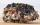On the 4-th stop take on 56 and take off 38 passengers. How many were added (write as positive number) or shrunk (write as negative number) the count of passengers?
3. Dividing by five and tenNumber 5040 divide by the number 5 and by number 10: a = 5040: 5 b = 5040: 10
4. Computer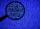A line of print on a computer contains 64 characters (letters, spacers or other chars). Find how many characters there are in 7 lines.
5. Book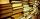Jitka read on holidays book that has 180 pages. In the first week read 45 pages. In the second week she read 15 pages more than the first week. How many pages left to read it yet?
6. FedorFedor stood in the small pool. Above the surface was a part of his body that was twice as long as part of his body underwater. What height does Fedor have, if his body part below the surface is 5 dm long?
7. Hotel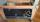The rooms in the mountain hotel are double and triple. Double rooms are 25 and triple are 17 more. How many rooms are there in this hotel?
8. Eq1Solve equation: 4(a-3)=3(2a-5)
9. Jan and DanJan and Dan had the same money. Jan bought 5 workbooks and left him 15 CZK. Dan 6 and left him nothing. How much money have in total?
10. If-then equationIf 5x - 17 = -x + 7, then x =
11. School marks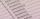Boris has a total of 22 marks. Ones have 3 times less than threes. There are two twos. How many has ones and threes?
12. CollectionMajka gave from her collection of calendars Hanke 15 calendars, Julke 6 calendars and Petke 10 calendars. Still remains 77 calendars. How many calendars had Majka in her collection at the beginning?
13. Tulips and daffodils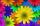Farm cultivated tulips and 211 units on 50 units more daffodils. How many spring flowers grown together?
14. Baking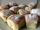There are 28 bunches, and son ate 1/2, dad ate four bunches. How many of them remain on the baking dishes?
15. Postal stamps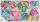Jano and Peter exchanged postal stamps. Jano gave Peter 32 stamps of the missile for 8 stamps with turtles. How good was Jano after this exchange (how many he has surplus in exchanged stamps)?
16. Fridays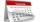Friday 13th is in 4 days. What day is today and what day it is?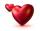Mom wants talk with dad 3 hours. But dad wants of 2 times more hours than mom ... How many hours wants dad talk with mom? ...